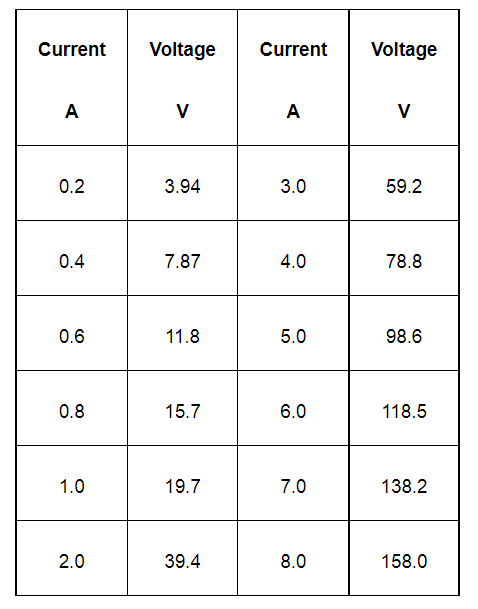# What conclusion can you draw from the following observations on a resistor made of alloy manganin?Question:

What conclusion can you draw from the following observations on a resistor made of alloy manganin?Solution:

It can be inferred from the given table that the ratio of voltage with current is a constant, which is equal to 19.7. Hence, manganin is an ohmic conductor i.e., the alloy obeys Ohm’s law. According to Ohm’s law, the ratio of voltage with current is the resistance of the conductor. Hence, the resistance of manganin is 19.7 Ω.